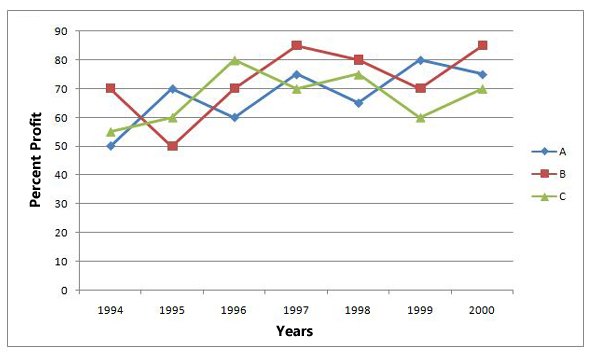## IBPS Clerk Quant Test 12

Instructions

Study the following graph carefully and answer the questions given below it.

Percent profit earned by three companies
A, B and C over the years 1994 to 2000
(Profit = Income - Expenditure)Question 1

Expenditure of the three Companies in the year 2000 were equal. What was the ratio of incomes of companies. A, B and C respectively in that year ?

Question 2

Expenditure of Company ’B’ in 1998 was Rs. 6.5 lakhs, which was equal to the income of Company ‘C’ in 1996. What was the ratio of income of COmpany ‘B’ in 1998 to the expenditure of Company ‘C’ in 1996 ?

Question 3

Income of Company ‘C’ in 1999 was Rs. 1,68,000. What was the expenditure in that year ?

Question 4

Income of Company ‘C’ in 1999 was Rs. 8.5 lakhs. What was its income in the year 2000 ?

Question 5

Expenditure of Company ‘B’ in 1996 is equal to the income of Company ‘A’ in 1998. What is the respective ratio of the expenditure of Companies B and A in 1996 and 1998 respectively ?